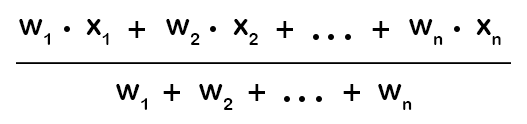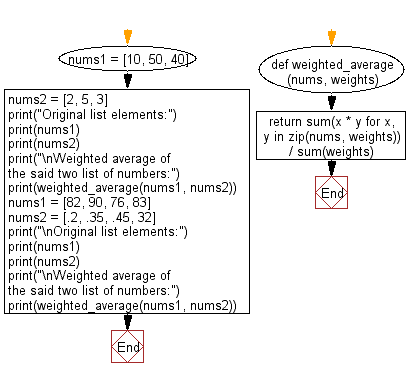﻿ Python: Find the weighted average of two or more numbers - w3resource# Python: Find the weighted average of two or more numbers

## Python List: Exercise - 254 with Solution

Write a Python program to get the weighted average of two or more numbers.

A weighted average is an average in which some of the items to be averaged are 'more important' or 'less important' than some of the others. The weights are (non-negative) numbers which measure the relative importance

For example, the weighted average of a list of numbers x1,…,xn with corresponding weights w1,…,wn isRef: mathinsight.org
• Use sum() to sum the products of the numbers by their weight and to sum the weights.
• Use zip() and a list comprehension to iterate over the pairs of values and weights.

Sample Solution:

Python Code:

``````def weighted_average(nums, weights):
return sum(x * y for x, y in zip(nums, weights)) / sum(weights)
nums1 = [10, 50, 40]
nums2 = [2, 5, 3]
print("Original list elements:")
print(nums1)
print(nums2)
print("\nWeighted average of the said two list of numbers:")
print(weighted_average(nums1, nums2))
nums1 = [82, 90, 76, 83]
nums2 = [.2, .35, .45, 32]
print("\nOriginal list elements:")
print(nums1)
print(nums2)
print("\nWeighted average of the said two list of numbers:")
print(weighted_average(nums1, nums2))
```
```

Sample Output:

```Original list elements:
[10, 50, 40]
[2, 5, 3]

Weighted average of the said two list of numbers:
39.0

Original list elements:
[82, 90, 76, 83]
[0.2, 0.35, 0.45, 32]

Weighted average of the said two list of numbers:
82.97272727272727
```

Flowchart:## Visualize Python code execution:

The following tool visualize what the computer is doing step-by-step as it executes the said program:

Python Code Editor:

Have another way to solve this solution? Contribute your code (and comments) through Disqus.

What is the difficulty level of this exercise?

Test your Python skills with w3resource's quiz

﻿

## Python: Tips of the Day

Floor Division:

When we speak of division we normally mean (/) float division operator, this will give a precise result in float format with decimals.

For a rounded integer result there is (//) floor division operator in Python. Floor division will only give integer results that are round numbers.

```print(1000 // 300)
print(1000 / 300)```

Output:

```3
3.3333333333333335```